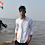# C Program to Sort Numbers in Ascending and Descending Order using Array

## Write a C program to sort numbers in ascending and descending order

Here in this article, I shared c program to sort numbers in ascending and descending order and also we use array concept to solve the problem.

Describe the program briefly? So, you easily understand the below program.
Firstly, I will clarify that I use the 30 elements array. so, you can't exceed this limit and if you want to exceed then you edit by your self. When you compile and execute the program it will ask for how many value of N, where N is the length of the array and then enter elements randomly in the array. I use switch case that will ask to enter your choice and show accordingly to it.

## C program to arrange numbers in ascending & descending order

### Source Code:

``````
#include<stdio.h>

int main()
{
int i,j,a,n,ch;
printf("Enter the Value of N \n");
scanf("%d",&n);
int number[n];
printf("Enter the Numbers \n");
for(i=0;i<n;i++)
{
scanf("%d",&number[i]);
}
while(1)
{
printf("Press 1. For ASCENDING ORDER\n");
printf("Press 2. For DESCENDING ORDER\n");
printf("Enter Your Choice:\n");
scanf("%d",&ch);
switch(ch)
{
case 1:  for(i=0;i<n;i++)
{
for(j=i+1;j<n;j++)
{
if(number[i]>number[j])
{
a=number[i];
number[i]=number[j];
number[j]=a;
}
}
}
printf("\nThe numbers arranged in ascending order are given below\n");
for(i=0;i<n;i++)
{
printf("%d\n",number[i]);
}
break;
case 2:
for(i=0;i<n;i++)
{
for(j=i+1;j<n;j++)
{
if(number[i]<number[j])
{
a=number[i];
number[i]=number[j];
number[j]=a;
}
}
}
printf("\nThe numbers arranged in descending order are given below\n");
for(i=0;i<n;i++)
{
printf("%d\n",number[i]);
}
break;
default:printf("\n INVALID CHOICE \n");
break;
}
}
return 0;
}
``````

Output:

```
Enter the value of N: 5```
```Enter the numbers
15
65
80
10
101```
```Press 1. For ASCENDING ORDER
Press 2. For DESCENDING ORDER
1
The numbers arranged in ascending order are given below
10
15
65
80
101```
```Press 1. For ASCENDING ORDER
Press 2. For DESCENDING ORDER
2
The numbers arranged in descending order are given below
101
80
65
15
10
```
```Press 1. For ASCENDING ORDER
Press 2. For DESCENDING ORDER
4
INVALID CHOICE
Press 1. For ASCENDING ORDER
Press 2. For DESCENDING ORDER

--------------------------------
```

In the above article, I shared C Program to Sort Numbers in Ascending and Descending Order using Array use loops and switch case, etc. If you know something new then please comment or send mail to featured and for contribution to codeamy.in and I also share c program soon.

Happy Coding!! 😊

If you have any doubts, Please let me know

1.1.Hi,

I hope you are doing good.
Please save file with .c extension and then try to run.
As I reviewed the source code it run successfully.

Thank You
codeamy.in

2.ive an error from the int number[n] part, or i need to install other plugins?